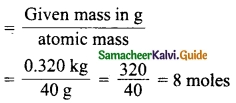Students can download 10th Science Chapter 7 Atoms and Molecules Questions and Answers, Notes, Samacheer Kalvi 10th Science Guide Pdf helps you to revise the complete Tamilnadu State Board New Syllabus, helps students complete homework assignments and to score high marks in board exams.

## Tamilnadu Samacheer Kalvi 10th Science Solutions Chapter 7 Atoms and Molecules

### Samacheer Kalvi 10th Science Atoms and Molecules Text Book Back Questions and Answers

Question 1.
Which of the following has the smallest mass?
(a) 6.023 × 1023 atoms of He
(b) 1 atom of He
(c) 2 g of He
(d) 1-mole atoms of He
(b) 1 atom of He

Question 2.
Which of the following is a triatomic molecule?
(a) Glucose
(b) Helium
(c) Carbon dioxide
(d) Hydrogen.
(c) Carbon dioxide
Hint:
(a) Glucose = C6H12O6 (Polyatomic molecule)
(b) Helium = He (Monoatomic molecule)
(c) Carbon dioxide = CO2 (Triatomic molecule)
(d) Hydrogen = H2 (Diatomic molecule)
So, (c) is the correct answer.

Question 3.
The volume occupied by 4.4 g of CO2 at S.T.P:
(a) 22.4 litre
(b) 2.24 litre
(c) 0.24 litre
(d) 0.1 litre
(b) 2.24 litreQuestion 4.
Mass of 1 mole of Nitrogen atom is _____.
(a) 28 amu
(b) 14 amu
(c) 28 g
(d) 14 g.
(b) 14 amu
Hint: Atomic mass of Nitrogen is 14.00674 grams. It is equal to 1 mole of Nitrogen atoms.

Question 5.
Which of the following represents 1 amu?
(a) Mass of a C – 12 atom
(b) Mass of a hydrogen atom
(c) 1/12 th of the mass of a C – 12 atom
(d) Mass of O – 16 atom
(c) 1/12 th of the mass of a C – 12 atom

Question 6.
Which of the following statement is incorrect?
(a) One gram of C – 12 contains Avogadro’s number of atoms.
(b) One mole of oxygen gas contains Avogadro’s number of molecules.
(c) One mole of hydrogen gas contains Avogadro’s number of atoms.
(d) One mole of electrons stands for 6.023 × 1023 electrons.
(a) One gram of C – 12 contains Avogadro’s number of atoms.
Hint: 12 g of Carbon contains 6.023 × 1023 atoms,
1 g of Carbon contain $$\frac{6.023 \times 10^{23}}{12}$$ = 5.018 × 1022 atoms and its is not Avogadro’s number of atoms.
So (a) is the incorrect statement.

Question 7.
The volume occupied by 1 mole of a diatomic gas at S.T.P is:
(a) 11.2 litre
(b) 5.6 litre
(c) 22.4 litre
(d) 44.8 litre
(c) 22.4 litre

Question 8.
In the nucleus of 20Ca40, there are
(a) 20 protons and 40 neutrons
(b) 20 protons and 20 neutrons
(c) 20 protons and 40 electrons
(d) 40 protons and 20 electrons
(b) 20 protons and 20 neutrons

Question 9.
The gram molecular mass of oxygen molecule is_____.
(a) 16 g
(b) 18 g
(c) 32 g
(d) 17 g.
(c) 32 g
Hint: By definition, the gram molecular mass of oxygen molecule O2 is 32 g.
So the answer (c) is correct.

Question 10.
1 mole of any substance contains molecules.
(a) 6.023 × 1023
(b) 6.023 × 10-23
(c) 3.0115 × 1023
(d) 12.046 × 1023
(a) 6.023 × 1023

II. Fill in the blanks:

1. Atoms of different elements having ……… mass number, but ………. atomic numbers are called isobars.
2. Atoms of different elements having same number of ………. are called isotones.
3. Atoms of one element can be transmuted into atoms of other element by ………….
4. The sum of the numbers of protons and neutrons of an atom is called its …………
5. Relative atomic mass is otherwise known as …………
6. The average atomic mass of hydrogen is ……….. amu.
7. If a molecule is made of similar kind of atoms, then it is called ……….. atomic molecule.
8. The number of atoms present in a molecule is called its ………….
9. One mole of any gas occupies ………… ml at S.T.P
10. Atomicity of phosphorous is …………
1. same, different
2. neutrons
3. artificial transmutation
4. mass number
5. standard atomic weight
6. 1.008
7. homo
8. atomicity
9. 22, 400
10. fourIII. Match the following: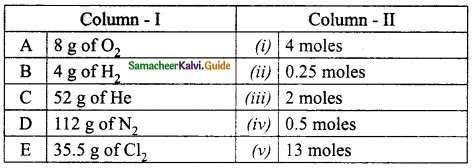A. (ii)
B. (iii)
C. (v)
D. (i)
E. (iv)

IV. True or False: (If false give the correct statement)

1. Two elements sometimes can form more than one compound.
2. Nobel gases are diatomic.
3. The gram atomic mass of an element has no unit.
4. 1 mole of Gold and Silver contain same number of atoms.
5. Molar mass of CO2 is 42 g.

1. True
2. False – Noble gases are Monoatomic.
3. False – The unit of gram atomic mass of an element is gram.
4. True
5. False – Molar mass of CO2 is 44 g.

V. Assertion and Reason:

Answer the following questions using the data given below:
Question 1.
Assertion: Atomic mass of aluminium is 27
Reason: An atom of aluminium is 27 times heavier than 1/12 th of the mass of the C-12 atom.
(a) Assertion and Reason are correct, Reason explains the Assertion.
(b) Assertion is correct, Reason is wrong.
(c) Assertion is wrong, Reason is correct.
(d) Assertion and Reason are correct, Reason doesn’t explains Assertion.
(a) Assertion and Reason are correct, Reason explains the Assertion.

Question 2.
Assertion: The Relative Molecular Mass of Chlorine is 35.5 a.m.u.
Reason: The natural abundance of Chlorine isotopes are not equal.
(a) Assertion and Reason are correct, Reason explains the Assertion.
(b) Assertion is correct, Reason is wrong.
(c) Assertion is wrong, Reason is correct.
(d) Assertion and Reason are correct, Reason doesn’t explains Assertion.
(c) Assertion is wrong, Reason is correct.

Question 1.
Define: Relative atomic mass.
Relative atomic mass of an element is the ratio between the average mass of its isotopes to $$\frac{1}{12^{th}}$$part of the mass of a carbon-12 atom. It is denoted as Ar.
[OR]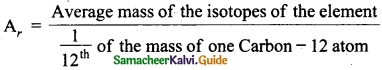Question 2.
Write the different types of isotopes of oxygen and its percentage abundance.
Oxygen has three stable isotopes. They are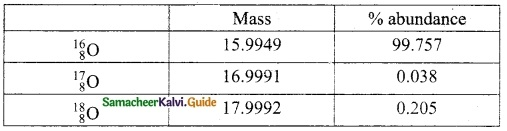Question 3.
Define Atomicity.
The number of atoms present in the molecule is called its ‘Atomicity’.

Question 4.
Give any two examples for heteroatomic molecules.
HI, HCl, CO, HBr, HF.Question 5.
What is Molar volume of a gas?
One mole of any gas occupies 22.4 litres.
(or)
22400 ml at S.T.R This volume is called as molar volume.

Question 6.
Find the percentage of nitrogen in ammonia.
Molar mass of NH3 = 1(14) + 3(1) = 17 g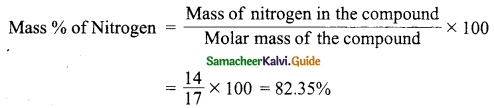Question 1.
Calculate the number of water molecule present in one drop of water which weighs 0.18 g.
The molecular mass of water (H2O) is 18.
18 g of water molecule = 1 mole.
0. 18 g of water = $$\frac{1}{18} \times 0.18$$ = 0.01 mole.
1 mole of water (Avogadro’s number) contains 6.023 × 1023 water molecules.
0. 01 mole of water contain $$\frac{6.023 \times 10^{23}}{1} \times 0.01$$ = 6.023 × 1021 molecules.

Question 2.
N2 + 3 H2 → 2 NH3
(The atomic mass of nitrogen is 14, and that of hydrogen is 1)
1 mole of nitrogen (……..g) +
3 moles of hydrogen (………g) →
2 moles of ammonia (………g)
1 mole of nitrogen (28 g) +
3 moles of hydrogen (6 g) →
2 moles of ammonia (34 g)

Question 3.
Calculate the number of moles in
(i) 27 g of Al;
(ii) 1.51 × 1023 molecules of NH4Cl.
(i) 27 g of Al
Given mass atomic mass = $$\frac{Given Mass}{Atomic Mass}$$ = $$\frac{27}{27}$$
= 1 mole

(ii) 1.51 x 1023 molecules of NH4Cl
Number of moles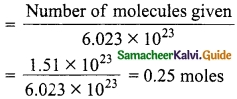Question 4.
Give the salient features of “Modern atomic theory”.
The salient features of “Modem atomic theory” are,

1. An atom is no longer indivisible.
2. Atoms of the same element may have different atomic mass.
3. Atoms of different elements may have the same atomic masses.
4. Atoms of one element can be transmuted into atoms of other elements. In other words, an atom is no longer indestructible.
5. Atoms may not always combine in a simple whole-number ratio.
6. Atom is the smallest particle that takes part in a chemical reaction.
7. The mass of an atom can be converted into energy [E = mc2].Question 5.
Derive the relationship between Relative molecular mass and Vapour density.
Relative molecular mass : The relative molecular mass of a gas or vapour is the ratio between the mass of one molecule of the gas or vapour to mass of one atom of hydrogen.
Vapour density : Vapour density is the ratio of the mass of certain volume of a gas or vapour, to the mass of an equal volume of hydrogen, measured under the same conditions of temperature and pressure.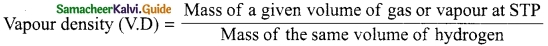According to Avogadro’s law equal volumes of all gases contain equal number of molecules.
Let the number of molecules in one volume = n, then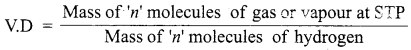When cancelling ‘n’ which is common at STP, we get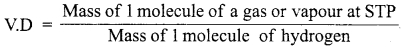Since hydrogen is diatomic,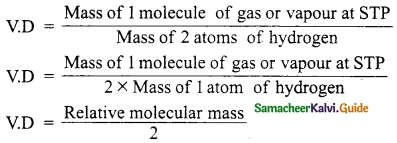2 × Vapour density = Relative Molecular mass of a gas
[OR]
Relative Molecular Mass = 2 × Vapour density

VIII. HOT Question:

Question 1.
Calcium carbonate is decomposed on heating in the following reaction
CaCO3 → CaO + CO2

1. How many moles of Calcium carbonate is involved in this reaction?
2. Calculate the gram molecular mass of calcium carbonate involved in this reaction.
3. How many moles of CO2 are there in this equation?

CaCO3 → CaO + CO2

1. 1 mole of CaCO3 is involved in this reaction.
2. Gram molecular mass of calcium carbonate
CaCO3 = (40 + 12 + 3 × 16) = 52 + 48 = 100 g
3. 1 mole of CO2 is in this equation.

IX. Solve the following problems:

Question 1.
How many grams are there in the following?
(i) 2 moles of a hydrogen molecule, H2
(ii) 3 moles of chlorine molecule, Cl2
(iii) 5 moles of sulphur molecule, S8
(iv) 4 moles of a phosphorous molecule, P4
Solution:
(i) 2 moles of a hydrogen molecule, H2
Mass of 1 mole of hydrogen molecule = 2 g
Mass of 2 moles of hydrogen molecule = 2 × 2 = 4 g.

(ii) 3 moles of chlorine molecule, Cl2
Mass of 1 mole of chlorine molecule = 71 g
Mass of 3 moles of chlorine molecules = 71 × 3 = 213 g.

(iii) 5 moles of sulphur molecule, S8
Mass of 1 mole of sulphur molecule = 32 g
Mass of 5 moles of sulphur molecules = 32 × 5 = 160 g.

(iv) 4 moles of the phosphorous molecule, P4
Mass of 1 mole of phosphorous molecule = 30.97 g
Mass of 4 moles of phosphorous molecules = 30.97 × 4 = 123.88 g.Question 2.
Calculate the % of each element in calcium carbonate. (Atomic mass: C – 12, O – 16, Ca – 40)
Formula to find % of each element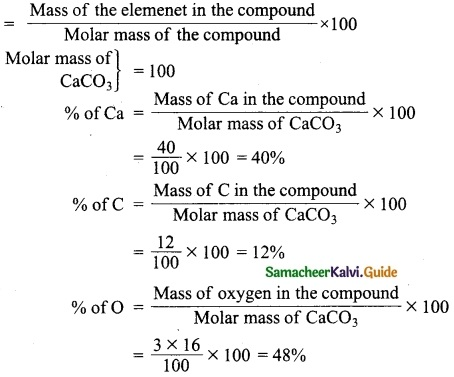Question 3.
Calculate the % of oxygen in Al2(SO4)3.
(Atomic mass: Al – 27, O – 16, S – 32)
Formula: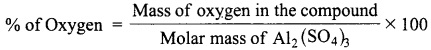Molar mass of Al2(SO4)3 = [2(Atomic mass of Al) + 3(Atomic mass of S) + 12(Atomic mass of O)]
= 2(27) + 3(32) + 12(16) = 342 g
% of Oxygen = $$\frac{12(16)}{342}$$ × 100 = 56.14%.

Question 4.
Calculate the % relative abundance of B – 10 and B – 11, if its average atomic mass is 10.804 amu.
% of relative abundance can be calculated by the formula.
Average atomic mass of the element
= Atomic mass of 1st isotope × abundance of 1st isotope + Atomic mass of 2nd isotope × abundance of 2nd isotope
∴ Average atomic mass of Boron
= Atomic mass of B – 0 × abundance of B -10 + Atomic mass of B – 11 × abundance of B – 11
Let the abundance of B – 10 be ‘x’ and B – 11 be (1 – x)
So, 10.804 = 10 × x + 11 (1 – x)
10.804 = 10x + 11 – 11x
x = 11 – 10.804
x = 0.196
1 -x = 1 – 0.196 = 0.804
Therefore % abundance of B – 10 is 19.6% and B – 11 is 80.4%
[OR]
Let the % of the isotope B – 10 = x
Then the % of the isotope B – 11 = 100 – x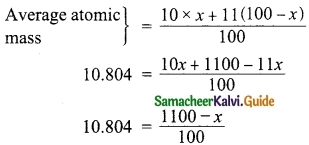1100 – x = 1080.4
x = 19.6
% abundance of B – 10 = 19.6%
% abundance of B – 11 = 80.4%

### Samacheer Kalvi 10th Science Atoms and Molecules Additional Important Questions and Answers

Question 1.
The first scientific theory of an atom was proposed by:
(a) Ruther Ford
(b) Newland
(c) John Dalton
(d) Neils Bohr
(c) John Dalton

Question 2.
Identify the pair that indicates isobars among the following _____.
(a) $$_{1} \mathrm{H}^{2},_{1} \mathrm{H}^{3}$$
(b) $$_{17} \mathrm{Cl}^{35},_{17} \mathrm{Cl}^{37}$$
(c) $$\text { (c) }_{18} \mathrm{Ar}^{40},_{18} \mathrm{Ca}^{40}$$
(d) $$_{6} \mathrm{C}^{13},_{7} \mathrm{N}^{14}$$.
(c) $$\text { (c) }_{18} \mathrm{Ar}^{40},_{18} \mathrm{Ca}^{40}$$Question 3.
Which one of the following represents 180 g of water?
(a) 5 moles of water
(b) 90 moles of water
(c) 6.023 × 1024 molecules of water
(d) 6.023 × 1022 molecules of water
(c) 6.023 × 1024 molecules of water

Question 4.
The isotope of Carbon-12 contains _____.
(a) 6 protons and 7 electrons
(b) 6 protons and 6 neutrons
(c) 12 protons and no neutrons
(d) 12 neutrons and no protons.
(b) 6 protons and 6 neutrons

Question 5.
Which contains the greatest number of moles of oxygen atoms?
(a) 1 mol of water
(b) 1 mole of NaOH
(c) 1 mole of Na2CO3
(d) 1 mole of CO
(c) 1 mole of Na2CO3

Question 6.
The mass of proton or neutron is approximately _____.
(a) 1 amu
(b) 1.609 × 10-19 g
(c) 1 g
(d) 6.023 × 10-23 g.
(a) 1 amu

Question 7.
The natural abundance of C-12 and C-13 are 98.90% and 1.10% respectively. The average atomic mass of carbon is:
(a) 12 amu
(b) 12.011 amu
(c) 14 amu
(d) 12.90 amu
(b) 12.011 amu

Question 8.
The relative atomic mass of magnesium-based on C – 12 scale is _____.
(a) 24 g
(b) 24
(c) 24 amu
(d) 24 kg
(b) 24

Question 9.
If 1.5 moles of oxygen combine with Al to form Al2O3, the mass of Al in g (atomic mass of Al = 27) used in the reaction is:
(a) 2.7
(b) 54
(c) 40.5
(d) 81
(b) 54

Question 10.
The atomicity of methane is:
(a) 5
(b) 4
(c) 3
(d) 6
(a) 5

Question 11.
Find the odd one out _____.
(a) $$_{8} \mathrm{O}^{16}$$
(b) $$_{8} \mathrm{O}^{17}$$
(c) $$_{6} \mathrm{O}^{12}$$
(d) $$_{8} \mathrm{O}^{18}$$.
(c) $$_{6} \mathrm{O}^{12}$$

Question 12.
The volume occupied by 3 moles of HCl gas at STP is:
(a) 22.4 L
(b) 44.8 L
(c) 2.24 L
(d) 67.2 L
(d) 67.2 LQuestion 13.
The mass percentage of hydrogen in ethane (C2H6) is:
(a) 25%
(b) 75%
(c) 80%
(d) 20%
(d) 20%

Question 14.
Which one of the following is a homo diatomic molecule?
(a) H2
(6) CO
(c) NO
(d) O3.
(a) H2

Question 15.
The percentage of nitrogen in urea is about:
(a) 38.4
(b) 46.6
(c) 59.1
(d) 61.3
(b) 46.6

Question 16.
Out of the following the largest number of atoms are contained in:
(a) 11 g of CO2
(b) 4 g of H2
(C) 5 g of NH3
(d) 8 g of SO2
(b) 4 g of H2

Question 17.
Which of the following is an example of a homo triatomic molecule?
(a) Phosphorous
(b) Sulphur
(c) Bromine
(d) Ozone.
(d) Ozone.

Question 18.
For the reaction A + 2B → C, 5 moles of A and 8 moles of B will produce:
(a) 5 moles of C
(b) 4 moles of C
(c) 8 moles of C
(d) 13 moles of C
(b) 4 moles of C

Question 19.
The vapour density of a gas is 32. Its relative molecular mass will be:
(a) 32
(b) 16
(c) 64
(d) 96
(c) 64

Question 20.
Find the odd one out _____.
(a) Silver
(b) Potassium
(c) Iron
(d) Phosphorous.
(d) Phosphorous.

II. Fill in the blanks.

1. The volume occupied by 16 g of oxygen is ………..
2. One mole of a triatomic gas contains ………… atoms.
3. Equal volume of all gases under the same conditions of temperature and pressure contain equal number of …………
4. The mass of an atom can be converted into energy by using the formula …………
5. The percentage composition is useful to determine the ………… formula and ………… formula.

1. 11.2 L
2. 3 × 6.023 × 1023
3. molecules
4. E = me²
5. empirical, molecularIII. Match the following:

Question 1.
Match the Column I with Column II.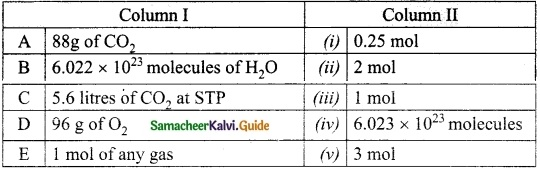A. (ii)
B. (iii)
C. (i)
D. (v)
E. (iv)

Question 2.
Match the Column I with Column II.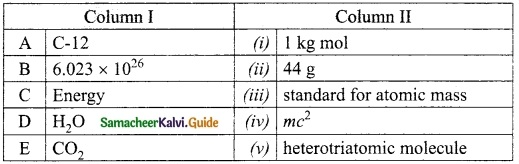A. (iii)
B. (i)
C. (iv)
D. (v)
E. (ii)

Question 3.
Match the Column I with Column II.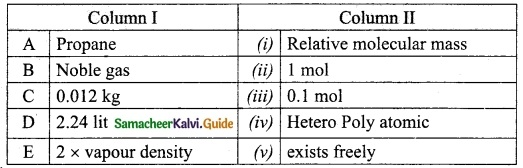A. (iv)
B. (v)
C. (ii)
D. (iii)
E. (i)

Question 4.
Match the Column I with Column II.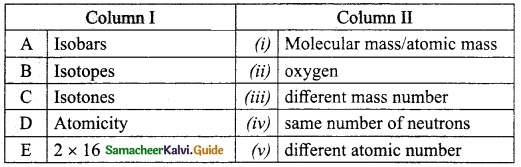A. (v)
B. (iii)
C. (iv)
D. (i)
E. (ii)

IV. True or False: (If false give the correct statement)

1. Atoms always combine in a simple whole number ratio.
2. 2 × RMM = VD
3. The average atomic mass of Beryllium is 9.012 because of the presence of isotopes.
4. The noble gases are diatomic.
5. The number of atoms present in one mole of phosphorus(P4) is 4 × 6.023 × 1023

1. False -Atoms may not combine always in a simple whole number ratio.
2. False – 2 × VD = RMM
3. True
4. False – The noble gases are mono atomic.
5. True

V. Assertion and Reason:

Answer the following questions using the data given below:
Question 1.
Assertion: The standard unit for expressing mass of atom is amu.
Reason: Atomic mass unit is one-twelth of the mass of a C-12 atom
(a) Assertion and Reason are correct, Reason explains the Assertion.
(b) Assertion is correct, Reason is wrong.
(c) Assertion is wrong, Reason is correct.
(d) Assertion and Reason are correct, Reason doesn’t explains Assertion.
(a) Assertion and Reason are correct, Reason explains the Assertion.

Question 2.
Assertion: The volume occupied by 44 g of CO2 is 22.4 L
Reason: The volume occupied by one mole of any gas is 22.4 L
(a) Assertion and Reason are correct, Reason explains the Assertion.
(b) Assertion is correct, Reason is wrong.
(c) Assertion is wrong, Reason is correct.
(d) Assertion and Reason are correct, Reason doesn’t explains Assertion.
(a) Assertion and Reason are correct, Reason explains the Assertion.Question 1.
Define vapour density.
Vapour density is the ratio of the mass of certain volume of a gas or vapour, to the mass of an equal volume of hydrogen measured under the same condition of temperature and pressure.
[OR]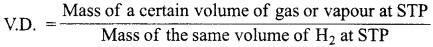Question 2.
What are isobars? Give an example.
Atoms of different elements that have same atomic masses but different atomic numbers are called isobars.
e.g., $$_{18} \mathrm{Ar}^{40}$$, $$_{20} \mathrm{Ca}^{40}$$.

Question 3.
Write the differences between an atom and a molecule.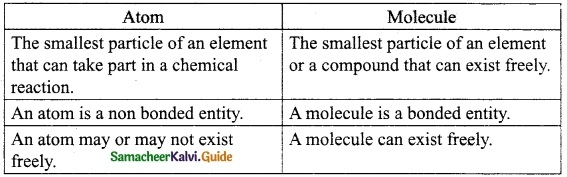Question 4.
What is artificial transmutation?
Atoms of one element can be transmuted into atoms of other elements. In other words, the atom is no longer indestructible. It is known as artificial transmutation.

Question 5.
Classify the following based on atomicity.
(i) Bromine
2 – Diatomic

(ii) Argon
1 – Monoatomic

(iii) Ozone
3 – Triatomic

(iv) Sulphur
8 – Polyatomic

Question 6.
Define atomic mass unit.
Atomic mass unit is one-twelfth of the mass of carbon – 12 atom, as an isotope of carbon which contains 6 protons and 6 neutrons. It is amu.

Question 1.
Explain how Avogadro hypothesis is used to derive the value of atomicity.
(i) The Avogadro’s law states that “equal volumes of all gases under similar conditions of temperature and pressure contain the equal number of molecules”.

(ii) Let us consider the reaction between hydrogen and chlorine to form hydrogen chloride gas.
H2 (g) + Cl2 (g) → 2HCl (g)
⇒ 1 volume + 1 volume → 2 volumes.

(iii) According to Avogadro’s law, 1 volume of any gas is occupied by “n” number of molecules,
“n” molecules + “n” molecules → “2n” molecules
If “n” = 1, then
1 molecule + 1 molecule → 2 molecules.
$$\frac { 1 }{ 2 }$$ molecule + $$\frac { 1 }{ 2 }$$ molecule → 1 molecule.

(iv) 1 molecule of hydrogen chloride gas is made up of $$\frac { 1 }{ 2 }$$ molecule of hydrogen and $$\frac { 1 }{ 2 }$$ molecule of chlorine.

(v) $$\frac { 1 }{ 2 }$$ molecule of hydrogen contains 1 atom.
So, 1 molecule of hydrogen contains 2 atoms.
So, hydrogen atomicity is 2. Similarly, chlorine atomicity is also 2.
So, H2 and Cl2 are diatomic molecules.Question 2.
Write a note on the following,
(i) Isotopeos
(ii) Isobars
(iii) Relative atomic mass.
(i) Isotopes : Atoms of same element with different mass number. Eg: 17Cl35, 17Cl37.
(ii) Isobars : Atoms of different elements with same mass number. Eg: 18Ar40, 20Ca40
(iii) Relative Atomic Mass (RAM) :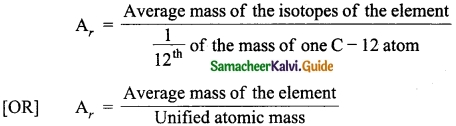Question 3.
Sodim bicarbonate breaks down on heating as follows:
2NaHCO3 → Na2CO3 + H2O + CO2
(Atomic mass of Na = 23, H = 1, C = 12, O = 16)
(i) How many moles of NaHCO3 are there in the equation?
2 moles.

(ii) What is the mass of CO2 produced in the equation?
44 g

(iii) How many moles of water molecules are produced in the equation?
1 mole.

(iv) What is the mass of NaHCO3 used in this equation?
= 2[23 + 1 + 12 + 3(16)]
= 2
= 168 g

(v) What is the volume occupied by CO2 in this equation?
22.4 lit.

VIII. Hot Questions

Question 1.
Why do we take an atomic mass of Carbon – 12 as standard?
Carbon – 12 is the standard while measuring the atomic masses. Because no other nuclides other than C – 12 have exactly whole-number masses in this scale. This is due to the different mass of neutrons and protons acting to change the total mass in nuclides with proton/neutron ratio other than 1 : 1 ratio of carbon – 12.

Question 2.
The cost of common salt (NaCl) is Rs 18 per kg. Calculate the cost of the salt per mole.
Gram molar mass of NaCl = 23 + 35.5
= 58.5 g
1000 g of NaCl costs = Rs 18
∴ 58.5 g of NaCl costs = $$\frac{18}{1000}$$ × 58.5
= Rs 1.053
The cost of one mole of NaCl = Rs 1.053

Question 3.
What will be the mass of one $$^{12} \mathbf{C}$$ atom in g?
1 mol of $$^{12} \mathbf{C}$$ atoms = 6.022 × 1023 atoms = 12 g
Thus, 6.022 × 1023 atoms of $$^{12} \mathbf{C}$$ have mass = 12 g
∴ 1 atom of $$^{12} \mathbf{C}$$ will have mass = $$\frac{12}{6.022 \times 10^{23}}$$ g = 1.9927 × 10-23 g.IX. Solve the following problems:

Question 1.
Calculate the average atomic mass of carbon, if the natural abundance of C – 12 and C – 13 are 98.90% and 1.10% respectively.
Solution:
Average atomic mass of carbon
$$=\left(12 \times \frac{98.9}{100}\right)+\left(13 \times \frac{1.1}{100}\right)$$
= (12 × 0.989) +(13 × 0.011)
= 11.868 + 0.143
= 12.011 amu.

Question 2.
Find how many moles are there in
(a) 98 g of H2SO4
(a) 98 g of H2SO4
GMM of H2SO4 = 2(1) + 32 + 4(16)
= 98 g
Number of moles = $$\frac{Given Mass}{Mol. Mass}$$
= $$\frac{98}{98}$$
= 1 mole

(b) 18.069 × 1023 atoms of calcium
Number of moles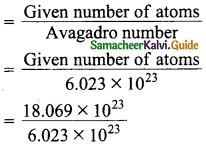= 3 moles

(c) 4.48 L of CO2
Number of moles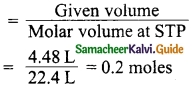Question 3.
Calculate the number of moles in
(i) 12.046 × 1023 atoms of copper
(ii) 27.95 g of iron
(iii) 1.51 × 1023 molecules of CO2
(i) 12.046 × 1023 atoms of copper
6.023 × 1023 atoms of copper = 1 mole
12.046 × 1023 atoms of copper = $$\frac{1 \times 12.046 \times 10^{23}}{6.023 \times 10^{23}}$$ = 2 moles of copper

(ii) 27.95 g of iron
55.9 g of iron = 1 mole
27.95 g of iron = $$\frac{1}{55.9}$$ × 27.95 = 0.5 mole of iron.

(iii) 1.51 × 1023 molecules of CO2
No of moles = $$\frac{\text { No. of molecules }}{\text { Avogadro number }}$$
= $$\frac{1.51 \times 10^{23}}{6.023 \times 10^{23}}$$
= 0.25 mole of CO2

Question 4.
Calculate the number of atoms of mercury present in 1 kg of Mercury. [Atomic mass of Hg = 200.6]
200.6 g of Hg contains 6.023 × 1023 Hg atoms
∴ 1 Kg of Hg will contain = $$\frac{6.023×10^{23}}{200.6}$$ × 1000
= 30.02 × 1023 Hg atoms

Question 5.
How many molecules are present in 7 × 10-3 m3 of NH3 at STP?
Molar volume = 22.4 dm³ = 2.24 × 10-2
2.24 × 10-2 m³ of NH3 at STP contains 6.023 × 1023 NH3 molecules.
:. 7 × 10-3 m³ of NH3 will contain
= $$\frac{6.023×10^{23}}{2.24×10^{-2}}$$ × 7 × 10-3
18.82 × 1022 NH3 molecule

Question 6.
What is the mass in grams of the following?
(a) 3 moles of NaOH
(b) 6.023 × 1022 atoms of Ca
(c) 224 L of CO2
Formula: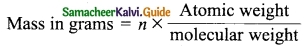(a) 3 moles of NaOH
Mass of 3 moles of NaOH = 3 × mol. mass of NaOH
GMM of NaOH = 23 + 16 + 1 = 40 g
Mass of 3 moles of NaOH = 3 × 40 = 120 g

(b) 6.023 × 1022 atoms of Ca = n × atomic mass of ca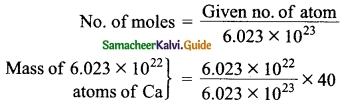= 4 g

(c) 224 L of CO2
Mass of 224 L of CO2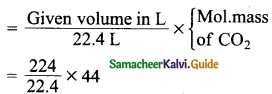= 10 × 44
= 440 gQuestion 7.
How many grams are therein:
(i) 5 moles of water
(ii) 2 moles of Ammonia
(iii) 2 moles of Glucose
Solution:
(i) 5 moles of water.
Mass of 1 mole of water (H2O) = 18 g (2 + 16)
Mass of 5 moles of H2O = 18 × 5 = 90 g.

(ii) 2 moles of ammonia.
Mass of 1 mole of ammonia (NH3) = 17 g (14 + 3)
Mass of 2 moles of ammonia = 17 × 2 = 34 g.

(iii) 2 moles of glucose.
Mass of 1 mole of glucose (C6H12O6) = 180 g (72 + 12 + 96)
Mass of 2 moles of glucose = 180 × 2 = 360 g.

Question 8.
Calculate tbe molar mass of the following compounds.
(a) Urea (NH2CONH2)
(b) Ethanol(C2H5OH);
(c) Boric acid (H3BO3)
[Atomic mass of N – 14, H – 1, C – 12, B – 11, O – 16]
(a) Urea (NH2CONH2) = 2(14) + 4(1) + 1(16) + 1(12)
= 28 + 4 + 16 + 12
= 60 g

(b) Ethanol(C2H5OH) = 2(12) + 6(1) + 1(16)
= 24 + 6 + 16 = 46 g

(c) Boric acid (H2BO3) = 3(1) + 1(11) + 3(16)
= 3 + 11 + 48
= 62 g

Question 9.
Mass of one atom of an element is 6.645 × 10-23 g. How many moles of element are there in 0.320 kg.